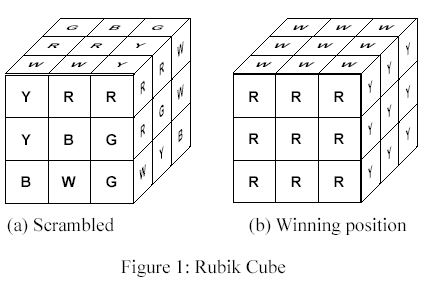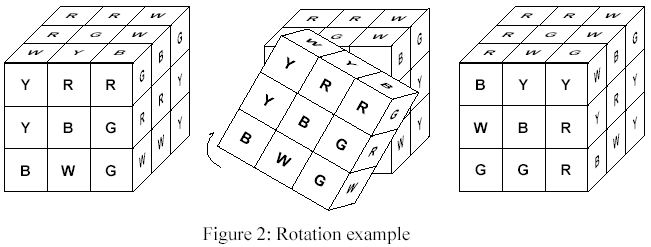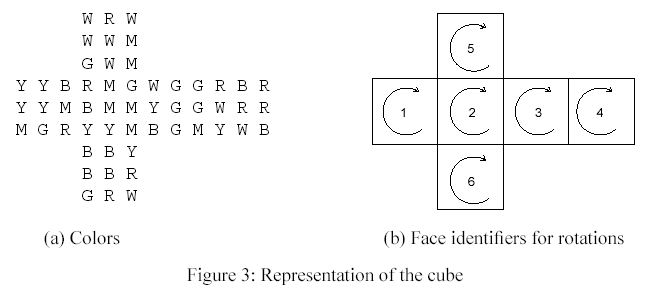Online JudgeProblem SetAuthorsOnline ContestsUser
Web Board
F.A.Qs
Statistical Charts
Problems
Submit Problem
Online Status
Prob.ID:
Register
Authors ranklist
Current Contest
Past Contests
Scheduled Contests
Award Contest
Register
Language:
Grandpa's Rubik Cube
 Time Limit: 1000MS Memory Limit: 10000K Total Submissions: 450 Accepted: 233

Description

A very well-known toy/pastime, called Rubik's cube, consists of a cube as shown in Figure 1a, where letters stand for colors (e.g. B for blue, R for red,...). The goal of the game is to rotate the faces of the cube in such a way that at the end each face has a different color, as shown in Figure 1b. Notice that,when a face is rotated, the configuration of colors in all the adjacent faces changes. Figure 2 illustrates a rotation of one of the faces. Given a scrambled configuration, reaching the final position can be quite challenging, as you may know.But your grandpa has many years of experience, and claims that, given any configuration of the Rubik cube, he can come up with a sequence of rotations leading to a winning configuration.In order to show all faces of the cube we shall represent the cube as in Figure 3a. The six colors are Yellow, Red, Blue, Green, White and Magenta (represented by their first letters).
You will be given an initial configuration and a list of rotations. A rotation will be represented by an integer number, indicating the face to be rotated and the direction of the rotation (a positive value means clockwise rotation, negative value means counter-clockwise rotation). Faces of the cube are numbered as shown in Figure 3b. You must write a program that checks whether the list of rotations will lead to a winning configuration.Input

The input contains several test cases. The first line of the input is an integer which indicates the number of tests. Each test description consists of ten lines of input. The first nine lines of a test will describe an initial configuration, in the format shown in Figure 3a. The next line will contain a list of rotations, ending with the value 0.

Output

For each test case your program should print one line. If your grandpa is correct, print "Yes,grandpa!", otherwise print "No, you are wrong!".

Sample Input

```3
G Y Y
G Y Y
G Y Y
W W W Y R R M M M G G B
W W W Y R R M M M G G B
W W W Y R R M M M G G B
R B B
R B B
R B B
-1 0
G Y Y
G Y Y
G Y Y
W W W Y R R M M M G G B
W M W Y R R M W M G G B
W W W Y R R M M M G G B
R B B
R B B
R B B
-1 0
M W M
W W G
W W Y
G Y Y M M B M B G W R B
B Y Y M M B M G G W R R
Y M G W B B R R G R R W
R Y Y
G B Y
R G B
+4 +6 -2 +3 -4 +2 -3 -6 0```

Sample Output

```Yes, grandpa!
No, you are wrong!
Yes, grandpa!```

Source

[Submit]   [Go Back]   [Status]   [Discuss]Home PageGo BackTo top